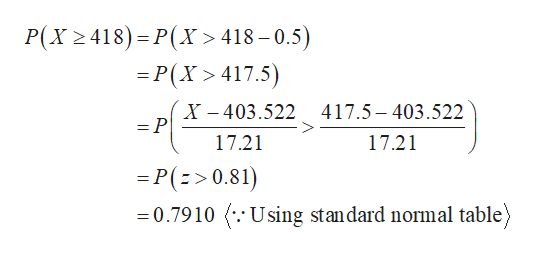# Assume that26.6%of people have sleepwalked. Assume that in a random sample of1517​adults,418have sleepwalked.a. Assuming that the rate of26.6%is​ correct, find the probability that418or more of the1517adults have sleepwalked.b. Is that result of418or more significantly​ high?c. What does the result suggest about the rate of26.6​%?

Question
Assume that
26.6%
of people have sleepwalked. Assume that in a random sample of
1517
418
have sleepwalked.
a. Assuming that the rate of
26.6%
is​ correct, find the probability that
418
or more of the
1517
b. Is that result of
418
or more significantly​ high?
c. What does the result suggest about the rate of
26.6​%?
check_circleExpert Solution
Step 1

It is given that p is 0.266 and n is 1,517.

Step 2

The mean and standard deviation using normal approximation to binomial are 403.522 (=1,517*0.266) and 17.210 (=sqrt(1,517*0.266*0.734))....help_outlineImage TranscriptioncloseP(X 418) P(X > 418-0.5) =P(X 417.5) X-403.522417.5-403.522 =P 17.21 17.21 -P(>0.81) 0.7910 .Using standard normal table fullscreen

### Want to see the full answer?

See Solution

#### Want to see this answer and more?

Solutions are written by subject experts who are available 24/7. Questions are typically answered within 1 hour*

See Solution
*Response times may vary by subject and question
Tagged in

### Statistics